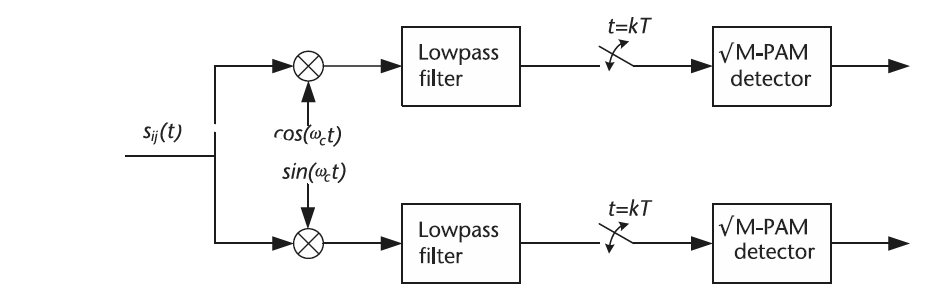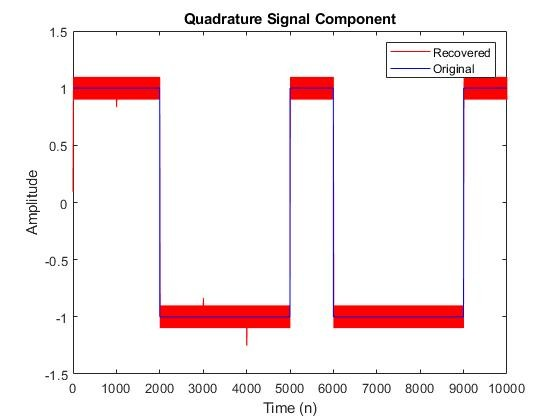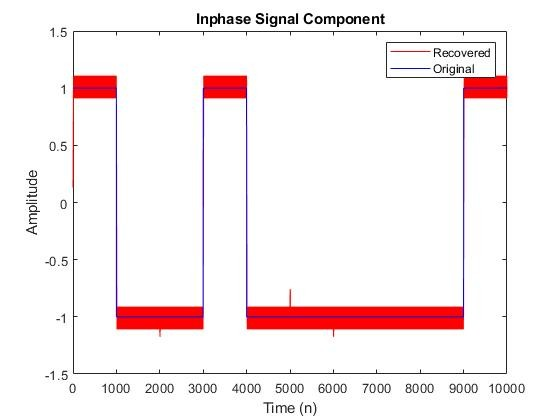QAM modulation is a two-dimensional signal modulation scheme as opposed to PAM modulation. The two dimensions of the QAM modulation; namely, the in-phase and quadrature components, are orthogonal to each other, which implies that one can essentially double the transmission data rate for free. Furthermore, rectangular QAM can be thought of as two orthogonal PAM signals being transmitted simultaneously.

Rectangular QAM is a popular modulation scheme due to its relatively simple receiver structure, as shown in the figure below.Code explanation: QAM modulation is a very useful technique for sending information efficiently within the same symbol period. As mentioned previously, it exploits both the in-phase and quadrature domains in order to transmit information in parallel down both channels orthogonally. In the MATLAB script above, we implement a QAM transmitter and receiver that takes binary vectors samp_I and samp_Q, modulates them to the in-phase and quadrature channels of a QAM transmission, and then extracts these binary vectors using QAM demodulation.

The output: